O Christmas tree,
O Christmas tree,
Last year a fractal made thee!
O Christmas tree,
O Christmas tree,
A heat map can display thee!

O tree of green, adorned with lights!
A trunk of brown, the rest is white.
O Christmas tree,
O Christmas tree,
A heat map can display thee!

Last week I showed how to use a heat map to visualize values in a matrix. To simplify the discussion, I allowed the ODS graphics in SAS to automatically choose colors based on the current ODS style. However, it is often useful to specify colors in order to improve the interpretability of a heat map. For example, in a recent article about how to specify colors in a mosaic plot, I used blue colors to represent negative values, white for nearly zero values, and red for positive values.

In the SAS Graph Template Language (GTL), you can use the DISCRETEATTRMAP statement and the DISCRETEATTRVAR statement to associate a color with each value in a matrix. Suppose that you want have a matrix with a small set of values, and you want to visualize the values by using the following colors:

• A missing value (.) is represented in the heat map by the color white
• The value 0 is represented by green
• The value 1 is represented by red
• The value 2 is represented by blue
• The value 3 is represented by yellow
• The value 4 is represented by brown

To illustrate assigning colors to values, I will create a "Christmas tree matrix." (You can think of each cell of the matrix as a "pixel" in the heat map.) The following program uses two helper functions (ROW and COL), which are explained in an article about how to fill specific cells of a matrix. The matrix is defined to have 100 rows and 101 columns. The trunk of the tree is 10% of its height. Within the "leafy" portion of the tree, I'll use the SAS/IML SAMPLE function to randomly place lights of three different colors.

```proc iml; /* define helper functions ROW and COL */ start row(x); /* return matrix m such that m[i,j] = i */ return( repeat( T(1:nrow(x)), 1, ncol(x) )); finish; start col(x); /* return matrix m such that m[i,j] = j */ return( repeat(1:ncol(x), nrow(x)) ); finish;   /* parameters for the tree dimensions */ h = 100; w = h+1; b = int(h/10); M = j(w, h, .); /* initialize h x w matrix to missing */ x = col(M); /* column numbers */ y = w + 1 - row(M); /* reverse Y axis */   /* define the leafy portion of the tree */ TreeIdx = loc(y>b & y<=2*x & y<=-2*x+2*h); /* a triangle */ M[TreeIdx] = 0;   /* place lights randomly within tree */ N = int(0.12*ncol(TreeIdx)); /* use 12% of tree area */ call randseed(1225); do i = 1 to 3; /* 3 colors */ idx = sample(TreeIdx, N, "WOR"); /* sample without replacement */ M[idx] = i; end;   /* define the trunk */ width = int(b/2); TrunkIdx = loc( y<= b & abs(x-nrow(M)/2)<width ); M[TrunkIdx] = 4;   /* write matrix in "long form" to SAS data set */ Row = row(M); /* row index */ Col = col(M); /* col index */ create Tree var {"Row" "Col" "M"}; append; close Tree; quit;```

As in last week's article, I'll use a GTL template to define the heat map. However, this time I'll associate specific colors to data values. The following template defines a green tree with a brown trunk that is decorated with red, blue, and yellow lights:

```proc template; define statgraph HeatmapTree; dynamic _X _Y _Z; begingraph; discreteattrmap name="christmastree"; value '.' / fillattrs=(color=WHITE); /* background color */ value '0' / fillattrs=(color=GREEN); /* tree color */ value '1' / fillattrs=(color=RED); /* ornament color 1 */ value '2' / fillattrs=(color=BLUE); /* ornament color 2 */ value '3' / fillattrs=(color=YELLOW); /* ornament color 3 */ value '4' / fillattrs=(color=BROWN); /* tree trunk color */ enddiscreteattrmap; discreteattrvar attrvar=Alias var=_Z attrmap="christmastree"; layout overlay / xaxisopts=(type=discrete display=none) yaxisopts=(type=discrete display=none reverse=true); heatmapparm x=_X y=_Y colorgroup=Alias / xbinaxis=false ybinaxis=false primary=true; endlayout; endgraph; end; run;   ods graphics / width=500 height=800; proc sgrender data=Tree template=HeatmapTree; dynamic _X="Col" _Y="Row" _Z="M"; run;```

The resulting heat map is shown at the beginning of this article.

You might not have many occasion where you need to use SAS to draw Christmas trees, but the same technique enables you to assign colors to any heat map that contains a small number of values. For matrices with a larger number of values, use the RANGEATTRMAP and RANGEATTRVAR statements to specify colors for a gradient color ramp, as shown in Chris Hemedinger's blog post.

This is my last post until after the Winter Break. I am taking a 10-day hiatus from blogging to recharge my mental batteries. If you've enjoyed reading any of my 100+ blog posts in 2013, please subscribe through an RSS feed and tell your friends. I look forward to learning and sharing more SAS programming techniques in 2014.Each year my siblings choose names for a Christmas gift exchange. It is not unusual for a sibling to pick her own name, whereupon the name is replaced into the hat and a new name is drawn. In fact, that "glitch" in the drawing process was a motivation for me to write a program that automates the Christmas gift exchange because my program ensure that no sibling gives to himself or to his spouse.

Last week I got an email from a reader whose wife had pulled her own name while participating in a Secret Santa gift exchange. There were 10 names in the hat, and the reader wanted to know the probability of someone pulling her own name. He wrote a SAS/IML simulation program that estimated the probability for various group sizes. His simulation indicated that for six or more people, there is only a 36.8% chance that no person pulls her own name. (Equivalently, someone needs to redraw 63.2% of the time.) What was surprising is that for moderate-sized groups, the probability is independent of the size of the group!

The reader wondered whether I could explain this strange number (0.368) and why the probability is independent of the group size.

### Permutations to the rescue

I was intrigued. Mathematically, pulling names from a hat is equivalent to a permutations of the numbers 1–n. There are two kinds of permutations. A "bad" permutation is one in which a number appears in its original position. (That is, a person draws her own name.) A "good" permutation has no number in its original position. The following SAS/IML statements generate three random permutations of the numbers 1–10. The first permutation is "bad" because the number 4 is in the 4th position and the number 7 is in the 7th position. The second permutation (not shown) is also bad. The third permutation is good because no number is in its original position.

```proc iml; call randseed(1); do i = 1 to 3; perm = ranperm(10); print perm; end;```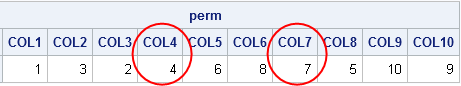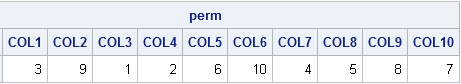### Complete permutations

A permutations such that no number appears in its original position is called a complete permutation or a derangement. For small sets, you can use the ALLPERM function in SAS/IML to generate all permutations. You can then count how many permutations are complete and print the proportion of derangement. The following program is a modification of the reader's program:

```N = T(2:10); /* size of group: 2--10 */ f = j(nrow(N),1); /* proportion for which person picks own name */ do i = 1 to nrow(N); /* for each group size */ p = allperm(N[i]); /* get all permutations */ nperms = nrow(p); ident = repeat(1:N[i], nperms); /* repeat 1,2,...,N for each row */ cnt = (p=ident)[,+]; /* count numbers in orig position */ f[i] = sum(cnt=0) / nperms; /* proportion of derangements */ end; print N f[L="Fraction of Derangements" f=7.5];```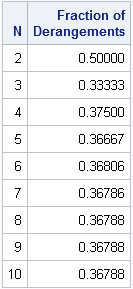### Counting derangements

As you can see, the proportion of derangements ("good draws") converges very quickly to 0.368. For a group of size six or more, this means that 63.2% of the time, someone will pull her own name (a bad draw).

It turns out that mathematicians have figured out how to count the number of derangements. Just as the number of permutations is called the factorial, the number of derangements is called the subfactorial. You can show that the number of derangements on n objects is given by [n! / e], where n! is the number of permutations, e ≈ 2.71828... is the base of the natural logarithm, and [⋅] is the nearest integer. In other words, the following SAS/IML function counts the number of complete permutations on n elements:
```start Subfactorial(n); return( round(fact(n)/exp(1)) ); finish;   N=2:10; s = Subfactorial(n); print (N//s)[r={"n" "Subfactorial(n)"}];```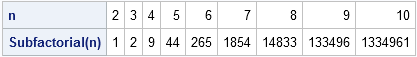By looking at the formula, you can see that the ratio of the number of derangements to the number of total permutations converges to 1/e ≈ 0.3678..., which explains the number that was computed by the earlier SAS/IML program.

So there you have it. If n ≥ 6 people randomly draw names out of a hat, there is approximately a 63.2% chance of someone pulling her own name. What is surprising is that this probability does not depend on the size of the group. Whether the group is six people or sixty people, the probability that someone draws her own name is approximately the same!No, I don't mean "rotation matrices," I mean rotating matrices. As in turning a matrix 90 degrees in a clockwise or counterclockwise direction.

I was reading a program written in MATLAB in which the programmer used a MATLAB function called ROT90, which rotates a matrix counterclockwise by multiples of 90 degrees. I thought it would be fun to implement that MATLAB function in the SAS/IML language.I wasn't sure of the most efficient way to implement the function. It seems like it might require writing a loop. But then I realized that rotating a matrix can be accomplished by using three simple "flip" operations: diagonal flips, up-down flips, and left-right flips. A flip across the diagonal is the matrix transpose operation. An up-down flip is equivalent to reversing the rows of a matrix. The left-right flip is accomplished by reversing the columns of a matrix. Each of these operations is easy to implement, and by composing two operations you can obtain a rotation of the elements of the matrix.

For example, the adjacent diagram shows that rotating a matrix by 90 degrees is equivalent to reversing its columns, followed by transposing the matrix. (Equivalently, you could transpose and then reverse the rows.) In the same way, you can rotate a matrix by 270 degrees by reversing the rows and then transposing. Lastly, you can rotate a matrix by 180 degrees by reversing the rows and the columns. Consequently, rotating a matrix is accomplished by the following one-line SAS/IML functions:

```proc iml; start Rot90(m); return( T(m[,ncol(m):1]) ); /* left-right flip, then transpose */ finish;   start Rot180(m); return( m[nrow(m):1,ncol(m):1] ); /* left-right flip, up-down flip */ finish;   start Rot270(m); return( T(m[nrow(m):1,]) ); /* up-down flip, then transpose */ finish;   m = shape(1:20,5); m90 = rot90(m); m180 = rot180(m); m270 = rot270(m); print m m90 m180 m270;```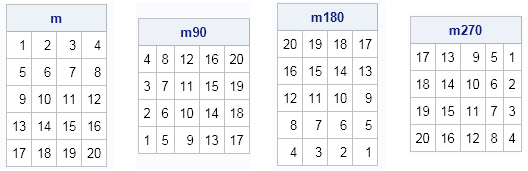The implementation of the functions shows that it is sometimes possible to generate a complicated transformation by considering products of simpler transformations. I have previously used the relationship between flips and rotations to demonstrate how to use matrix operations to extend the life of your bed mattress. Whether you are rotating mattresses or matrices, the task is made easier by using geometry and the SAS/IML language.While walking in the woods, a statistician named Goldilocks wanders into a cottage and discovers three bears. The bears, being hungry, threaten to eat the young lady, but Goldilocks begs them to give her a chance to win her freedom.

The bears agree. While Mama Bear and Papa Bear block Goldilocks' view, Baby Bear tosses a coin 30 times and records the results. He then makes up two other (fake) sequences of heads and tails, and gives Goldilocks a piece of paper that shows all three sequences. Papa Bear growls, "If you can determine which sequence came from the real coin toss, we will let you go. Otherwise we will eat you for dinner, for I have grown tired of porridge."

Here are the three sequences of heads (H) and tails (T) that the bears present to Goldilocks. Each of the sequences contain 16 heads and 14 tails.

1. H H H H H H H H H H H H H H H H T T T T T T T T T T T T T T
2. H T H T H T H T H T H T H T H T H T H T H T H T H T H T H H
3. H T T H H H T T T T T T T H H H T H T H H H T H H H T H T H

Goldilocks studies the three sequences and tells Papa Bear:

The first sequence is "too hot." It contains 16 heads followed by 14 tails. I would not expect such long sequences of heads and tails. Similarly, the second sequence is "too cold." It alternates between heads and tails like clockwork. The third sequence is "just right." It matches my intuitive notion of a random sequence of two categories: many short subsequences interlaced with a few longer subsequences. I think that the third sequence is real.

She had chosen correctly. The three bears, impressed by her statistical knowledge, set Goldilocks free and—once again—reluctantly ate porridge for dinner.

### Implementing the "runs test" in SAS

Goldilocks reasoned well. If she had brought along her laptop with SAS software on it, she could have used statistics to analyze the data.

You can quantify Goldilocks' intuitive notions by defining a run as a sequence of consecutive trials that result in the same value. The first sequence has two runs: a run of heads followed by a run of tails. The second sequence has 29 runs. The third sequence has 15 runs: eight runs of heads and seven runs of tails.

It turns out that you can calculate the expected number of runs in a random sequence that has n heads and m tails. The expected number of runs is E(R) = 2nm / (n+m) + 1. The three sequences have n=16 heads and m=14 tails, so the expected number of runs is 15.9. So Goldilocks' intuition was correct: the first sequence does not have enough runs, whereas the second has too many. The third sequence has 15 runs, which is close to the expected value.

You can use these facts to construct a statistical test for the randomness of a two-category sequence, based on the number of runs in the sequence. This is called the runs test, or the Wald-Wolfowitz test for randomness. There is a SAS Knowledge Base article that describes how to implement the runs test in SAS by using the DATA step. However, the DATA step implementation is longer and more complicated than an analogous implementation in the SAS/IML language.

To simplify counting the number of runs, recode the two categories (heads and tails) as –1 and +1. Then a "run" is a sequence of consecutive values that have the same sign. I have previously blogged about how to use the DIF-SIGN technique to detect when terms in a sequence switch signs. In the following program, that technique is used to count the number of runs in a sequence.

```proc iml; /* Implement the Wald-Wolfowitz test (or "runs test"). The SEQ vector is assumed to contain two groups (char or numeric). Compare with Base SAS version: http://support.sas.com/kb/33/092.html */ start RunsTest(seq); u = unique(seq); /* find unique values (should be two) */ d = j(nrow(seq)*ncol(seq),1, 1);/* recode as vector of -1, +1 */ d[loc(seq=u)] = -1; n = sum(d>0); /* count number of +1s */ m = sum(d<0); /* count number of -1s */   /* Use DIF-SIGN trick to count the number of runs */ dif = dif(sign(d)); /* difference in signs */ dif = choose(d<0, -2, 2); /* replace first obs */ R = sum(dif=2 | dif=-2); /* R = observed number of runs */   mu = 2*n*m / (n+m) + 1; /* E(R)=expected number of runs, given n, m */ var = 2*n*m*(2*n*m-(n+m)) / ((n+m)##2*(n+m-1)); /* var(R) */ sigma = sqrt(var); /* StdDev(R) */   /* R is asymptotically normally distributed. Compute test statistic. */ if (n+m)>50 then Z = (R-mu) /sigma; else if R-mu < 0 then Z = (R-mu+0.5)/sigma; /* for small samples, apply */ else Z = (R-mu-0.5)/sigma; /* continuity correction */   return(Z || 2*(1-cdf("Normal", abs(Z)))); /* (Z, two-sided p-value) */ finish;   flips = {H T T H H H T T T T T T T H H H T H T H H H T H H H T H T H}`; Test = RunsTest(flips); print Test[c={"Runs Statistic" "p-value"} L="Runs Test, two-sided"];```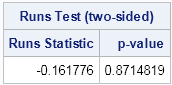The output of the RunsTest module is a two-element vector. The first element is the test statistic and the second element is the two-side p-value. Running the test on Baby Bear's coin-toss sequence produces a large p-value, which means that Goldilocks would accept the null hypothesis that the sequence of coin tosses is random. You can run the test for the other two sequences ("too hot" and "too cold") and see that both of them produce tiny p-values. If Goldilocks had implemented the runs test, she would have concluded that the made-up sequences are unlikely to be random sequence of coin flips.

I'll conclude with a few thoughts:

• The SAS/IML language is useful for implementing statistical methods, such as the runs test, that are not included in any SAS procedure.
• The SAS/IML language is compact. For this example, the SAS/IML program contains about 15 lines, versus 65 lines for the DATA step code.
• When I first thought about this problem, I thought I would need to use a loop to count the number of runs in a sequence. However, by recoding the data as +/–1, I was able to vectorize the computation by using the DIF-SIGN technique.
• Goldilocks was fortunate to know statistics.Editor's Note: My 8th grade son, David, created a poster that he submitted to the 2013 ASA Poster Competition. The competition encourages students to display "two or more related graphics that summarize a set of data, look at the data from different points of view, and answer specific questions about the data." In this guest blog, David explains his experiment and analysis. All prize-winning posters are featured in the August 2013 issue of Amstat News.
-- Rick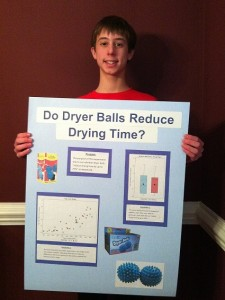Hi! My name is David Wicklin. This blog post describes a research project and statistical graphs that I created for the 2013 ASA Poster Competition.

My poster began one day when my sister saw a small box on a store shelf that contained two spiked plastic balls. The balls were about the size of tennis balls. The box said that you should put these "dryer balls" in your dryer with a load of wet laundry. The box claimed that the balls would "reduce drying time by up to 25%." I was skeptical. Could putting plastic balls in the dryer really reduce drying time? I was looking for a project for my school science fair, so I decided to conduct an experiment to find out.

My family's dryer has a "dampness sensor" that beeps when a load of laundry is dry. I decided to use this feature and a stop watch to determine the drying time for a load of laundry. For half the loads, I'd toss in two dryer balls. For others I would not.

### Experimental Method

The first issue was how to design the experiment. One option was a controlled experiment in which I would select clothes of different materials (jeans, sweatshirts, tee shirts, and so forth) and different quantities (small, medium, and large loads). I would wash and dry each load twice: once with dryer balls and once without. That would make comparing drying times easy because the only varying factor would be the dryer balls; the clothes would be exactly the same.

However, from a practical point of view, a controlled experiment was not ideal. It would require wasting time and energy to wash and dry items twice. Plus, my family had real laundry that needed washing and drying! My dad suggested that we wash the regular family clothes and compare the mean drying times. If I washed enough laundry, I should be able to account for the random differences between the loads, such as the materials being dried. I would weigh the clothes to keep track of the size of the load.

I got my mom to agree to let me dry our family's laundry over the next few months. As you might suspect, she was very much in favor of this activity! I dried a total of 40 loads of laundry. For each load, I weighed the wet clothes by using a spring scale. For 20 randomly selected loads, I added two dryer balls to the dryer. The other 20 loads had no balls, just the clothes. Each load was dried using the same dryer setting. All types of clothing were used to randomize the samples. For each load, I recorded the weight and the drying time in a notebook.

### Analyzing and graphing the data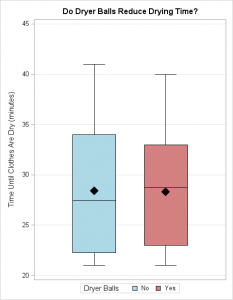I created two different graphs. The first is a side-by-side box and whisker diagram. This shows that the average drying times are not separated by much. For loads without dryer balls, the average drying time was 28.4 minutes. The median drying time was 27.5 minutes, and the first and third quartiles were 22.25 and 34 minutes. For loads with dryer balls added, the average drying time was 28.3 minutes. The median drying time was 28.75 minutes, and the first and third quartiles were 23 and 33 minutes.

The box and whisker diagram shows that it does not matter if you add dryer balls or not. If dryer balls really "reduce drying time by up to 25%," I would expect the red box plot to be shifted down by 5–8 minutes as compared to the blue box. It isn't. The two boxes are essentially the same.

But maybe dryer balls only "work" for big loads (or small loads). To find out, I created a scatter plot that displays the drying time and the weight of the wet clothes. I plotted blue squares for drying times that did not use dryer balls. I plotted red circles for times that included dryer balls.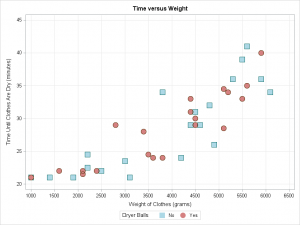The scatter plot shows that dryer balls do not affect drying time whether it is a heavy load or a light one. For light loads, it takes about 22 minutes to dry the laundry. For heavy loads it takes about 35 minutes. The red and blue markers are mixed together. If dryer balls actually reduce drying time, I would expect the red markers to be mostly below the blue markers.

The scatter plot enables me to estimate how long it will take to dry clothes, which is pretty cool! If I know that a load of laundry weighs 4 kg, I can predict that the load will take about 27 minutes to dry. I would be very confident to predict that the load will dry between 24 and 34 minutes.

The drying time obviously increases with the weight of the wet clothes. However, it is not clear if the drying time increases in a straight line or maybe a curve.

### Conclusions and Reflections

A limitation of my experiment is that I only used one washer and dryer. Our family's dryer is fairly new and our washer is "high efficiency," which means that it spins out a lot of the water. I have not done the experiment with an older, conventional, washer.

Based on my results, I conclude that the mean drying time does not depend on whether dryer balls are used. In particular, dryer balls do not “reduce drying time by up to 25%.” The observed difference was about 1%, but that could be due to randomness. The time required to dry clothes depends on the weight of the wet clothes, but using dryer balls does not make any difference in the time it takes to dry the clothes, whether the load is small or large.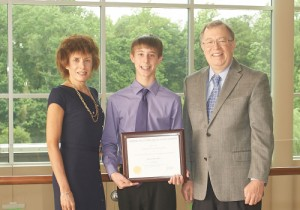I am very happy to report that this poster was awarded second place in the nation for the 7–9 grade level! I am excited to be chosen for this honor. I have to thank my dad because without him I never would have been motivated enough to make this poster or record and plot 40 loads of laundry.

I got presented the award at a very special ceremony that featured the 2013 president of the ASA, Marie Davidian, a professor in the Statistics Department at NC State University. Also at the ceremony was the 2012 president, Bob Rodriguez, a senior director at SAS. They gave me a plaque and I told them all about my project. They were really nice and very friendly. It really meant a lot to me that they would take time out of their busy day to show interest in my poster and to encourage me to pursue science and math in the future.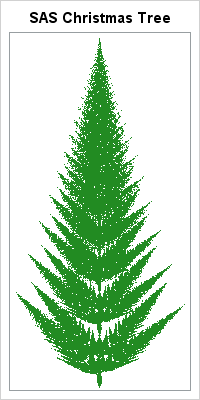In my previous post, I described how to implement an iterated function system (IFS) in the SAS/IML language to draw fractals. I used the famous Barnsley fern example to illustrate the technique. At the end of the article I issued a challenge: can you construct an IFS whose fractal attractor looks like a Christmas tree?

I started my attempt by "straightening" the fern example, but it ended up looking like a "fishbone fern" instead of an evergreen tree. I decided to abandon the four-function IFS and adopt a seven-function system. In addition to "left" and "right" branches, I introduced transformations for "left diagonal" and "right diagonal" branches. I also played with the relative probabilities and the translation parameters to get the right "fill" for the tree.

Eventually I constructed the following program, which implements an IFS that creates the "SAS Christmas tree" that is shown at the left. I'm not 100% satisfied with it, but as Linus says in A Charlie Brown Christmas, it's not "such a bad little tree."

```proc iml; /* For an explanation of how to construct an iterated function system in SAS, see http://blogs.sas.com/content/iml/2012/12/12/iterated-function-systems-and-barnsleys-fern-in-sas/ */ /* Each row is a 2x2 linear transformation */ /* Christmas tree */ L = {0.03 0 0 0.1, 0.85 0.00 0.00 0.85, 0.8 0.00 0.00 0.8, 0.2 -0.08 0.15 0.22, -0.2 0.08 0.15 0.22, 0.25 -0.1 0.12 0.25, -0.2 0.1 0.12 0.2}; /* ... and each row is a translation vector */ B = {0 0, 0 1.5, 0 1.5, 0 0.85, 0 0.85, 0 0.3, 0 0.4 }; prob = { 0.02 0.6 0.1 0.07 0.07 0.07 0.07}; L = L`; B = B`; /* For convenience, transpose the L and B matrices */   /* Iterate the discrete stochastic map */ N = 1e5; /* number of iterations */ x = j(2,N); k = j(N,1); x[,1] = {0, 2}; /* initial point */ call randgen(k, "Table", prob); /* values 1-7 */   do i = 2 to N; x[,i] = shape(L[,k[i]], 2)*x[,i-1] + B[,k[i]]; /* iterate */ end;   /* Plot the iteration history */ y = x`; create IFS from y[c={"x" "y"}]; append from y; close IFS; quit;   /* basic IFS Christmas Tree */ ods graphics / width=200px height=400px; proc sgplot data=IFS; title "SAS Christmas Tree"; scatter x=x y=y / markerattrs=(size=1 color=ForestGreen); yaxis display=none; xaxis display=none; run;```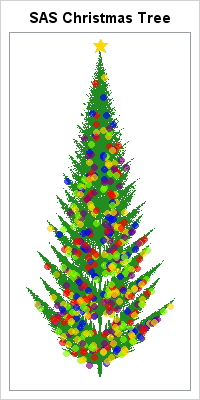As I looked at the tree, however, I decided that it seemed rather bare. It seemed to "need a little love," so I decided to add some brightly colored, ornamental, decorations. I took a random sample of points on the attractor and drew balls at those points. I also added a star on top, all courtesy of PROC SGPLOT. You can download the complete program that creates the final image, which is shown at left. I used a few programming tricks, such as a data attribute map to assign customized, bright, colors for the ornaments.

So now it's your turn! In the comments, submit or link to a SAS program that creates a Christmas tree, a snowflake, or some other seasonal image. Be creative! My colleague, Robert Allison, has already posted a few whimsical SAS graphs for the holidays.

As for me, I'm finished blogging until the New Year. However, I'm not exactly taking a vacation from SAS programming because I'll be working to finish my forthcoming book, Simulating Data with SAS.

Thank you, readers, for an amazing year of blogging, interacting, and sharing ideas in 2012. Thanks to you, this blog has become the most widely-read blog at SAS!

tags: Just for FunIn my previous post, I described how to implement an iterated function system (IFS) in the SAS/IML language to draw fractals. I used the famous Barnsley fern example to illustrate the technique. At the end of the article I issued a challenge: can you construct an IFS whose fractal attractor looks like a Christmas tree?

I started my attempt by "straightening" the fern example, but it ended up looking like a "fishbone fern" instead of an evergreen tree. I decided to abandon the four-function IFS and adopt a seven-function system. In addition to "left" and "right" branches, I introduced transformations for "left diagonal" and "right diagonal" branches. I also played with the relative probabilities and the translation parameters to get the right "fill" for the tree.

Eventually I constructed the following program, which implements an IFS that creates the "SAS Christmas tree" that is shown at the left. I'm not 100% satisfied with it, but as Linus says in A Charlie Brown Christmas, it's not "such a bad little tree."

```proc iml; /* For an explanation of how to construct an iterated function system in SAS, see http://blogs.sas.com/content/iml/2012/12/12/iterated-function-systems-and-barnsleys-fern-in-sas/ */ /* Each row is a 2x2 linear transformation */ /* Christmas tree */ L = {0.03 0 0 0.1, 0.85 0.00 0.00 0.85, 0.8 0.00 0.00 0.8, 0.2 -0.08 0.15 0.22, -0.2 0.08 0.15 0.22, 0.25 -0.1 0.12 0.25, -0.2 0.1 0.12 0.2}; /* ... and each row is a translation vector */ B = {0 0, 0 1.5, 0 1.5, 0 0.85, 0 0.85, 0 0.3, 0 0.4 }; prob = { 0.02 0.6 0.1 0.07 0.07 0.07 0.07}; L = L`; B = B`; /* For convenience, transpose the L and B matrices */   /* Iterate the discrete stochastic map */ N = 1e5; /* number of iterations */ x = j(2,N); k = j(N,1); x[,1] = {0, 2}; /* initial point */ call randgen(k, "Table", prob); /* values 1-7 */   do i = 2 to N; x[,i] = shape(L[,k[i]], 2)*x[,i-1] + B[,k[i]]; /* iterate */ end;   /* Plot the iteration history */ y = x`; create IFS from y[c={"x" "y"}]; append from y; close IFS; quit;   /* basic IFS Christmas Tree */ ods graphics / width=200px height=400px; proc sgplot data=IFS; title "SAS Christmas Tree"; scatter x=x y=y / markerattrs=(size=1 color=ForestGreen); yaxis display=none; xaxis display=none; run;```As I looked at the tree, however, I decided that it seemed rather bare. It seemed to "need a little love," so I decided to add some brightly colored, ornamental, decorations. I took a random sample of points on the attractor and drew balls at those points. I also added a star on top, all courtesy of PROC SGPLOT. You can download the complete program that creates the final image, which is shown at left. I used a few programming tricks, such as a data attribute map to assign customized, bright, colors for the ornaments.

So now it's your turn! In the comments, submit or link to a SAS program that creates a Christmas tree, a snowflake, or some other seasonal image. Be creative! My colleague, Robert Allison, has already posted a few whimsical SAS graphs for the holidays.

As for me, I'm finished blogging until the New Year. However, I'm not exactly taking a vacation from SAS programming because I'll be working to finish my forthcoming book, Simulating Data with SAS.

Thank you, readers, for an amazing year of blogging, interacting, and sharing ideas in 2012. Thanks to you, this blog has become the most widely-read blog at SAS!

tags: Just for FunFractals. If you grew up in the 1980s or '90s and were interested in math and computers, chances are you played with computer generation of fractals. Who knows how many hours of computer time was spent computing Mandelbrot sets and Julia sets to ever-increasing resolutions?

When I was a kid, I realized that there were two kinds of fractals: the deterministic ones, such as the Mandelbrot set, and the probabilistic ones, such as the Barnsley fern. I spent most of my time generating the deterministic fractals, because they were simpler to understand for someone who knew little about probability or linear transformations.

Yet, I was always fascinated by how to create objects by using Michael Barnsley's iterated function systems (IFS). You can construct an IFS by defining some number of affine planar transformations, selecting one at random with some probability, and applying it to an initial point. By iteratively selecting and applying these affine transformations, the trajectory of the point traces out some fractal shape, such as the fern shown at the left. Now that I am older and know a little bit about probability and linear transformations, let's investigate how to construct Barnsley's fern in the SAS/IML matrix language. I invite DATA step programmers to create a similar program by using the SAS DATA step.

### Construct Barnsley's Fern in SAS

Before I began programming the IFS, I reminded myself how to construct an iterated function system. Take a few minutes to read about the underlying mathematics. You are then ready to construct the IFS for Barnsley's fern:

1. Create four affine transformations in the plane. Each affine transformation is of the form T(x) = A*x + b, where A is a 2x2 matrix, b is a 2x1 vector, and x is a 2x1 vector that represents a point in the plane.
2. Specify the probability of selecting each of the four transformations.
3. Iterate the discrete stochastic map by selecting an initial point and repeatedly doing the following a large number of times:
• Choose one of the transformations at random, using the given (unequal) probabilities.
• Apply the chosen transformation to the current point to obtain a new (image) point.
4. Plot the trajectory (or orbit) of the initial point.
Under certain conditions on the transformations (they need to be contracting), the orbit of the initial point is attracted to a fractal set.

The following SAS/IML program implements an iterated function system for creating Barnsley's fern. The SGPLOT procedure is used to plot the trajectory. The main programming details are that the RANDGEN subroutine is called to generate random numbers from the "Table" distribution, and the SHAPE function is used to convert a row of coefficients into a 2x2 matrix. The SAS/IML matrix-vector operations are used to apply each affine transformation.

```/* http://en.wikipedia.org/wiki/Barnsley_fern The Barnsley fern is created with four affine transformations: T1(x,y) = [ 0 0 ] * [x] with prob=0.01 [ 0 0.16] [y]   T2(x,y) = [ 0.85 0.04] * [x] + [0 ] with prob=0.85 [-0.04 0.85] [y] [1.6]   T3(x,y) = [ 0.2 -0.26] * [x] + [0 ] with prob=0.07 [ 0.23 0.22] [y] [1.6]   T4(x,y) = [-0.15 0.28] * [x] + [0 ] with prob=0.07 [ 0.26 0.24] [y] [0.44] */ proc iml; /* 1. Each row is a 2x2 linear transformation */ L = {0 0 0 0.16, /* L1 */ 0.85 0.04 -0.04 0.85, /* L2 */ 0.2 -0.26 0.23 0.22, /* L3 */ -0.15 0.28 0.26 0.24}; /* L4 */   /* ... and each row is a translation vector */ B = {0 0, /* B1 */ 0 1.6, /* B2 */ 0 1.6, /* B3 */ 0 0.44}; /* B4 */ /* For convenience, transpose the L and B matrices */ L = L`; B = B`;   /* 2. The transformations are of the form T(x) = A*x + b where A and b are chosen randomly from the columns of L and B. Specify probability of each transformation. */ prob = {0.01 0.85 0.07 0.07};   /* 3. iterate the discrete stochastic map */ N = 1e5; /* number of iterations */ x = j(2,N); x[,1] = {0, 2}; /* initial point */   do i = 2 to N; call randgen(k, "Table", prob); /* k is a number 1,2,3 or 4 */ x[,i] = shape(L[,k], 2)*x[,i-1] + B[,k]; /* apply transformation to x[i-1] */ end;   /* 4. plot the iteration history */ y = x`; create IFS from y[c={"x" "y"}]; append from y; close IFS; quit;   ods graphics / width=200px height=400px; proc sgplot data=IFS; title "Barnsley Fern"; scatter x=x y=y / markerattrs=(size=1); yaxis display=none; xaxis display=none; run;```

The algorithm creates the image that appears at the beginning of this article.

### The holiday challenge: Construct an IFS for a Christmas tree

The cool thing about iterated function systems is that the affine transformations and the probabilities are actually parameters that you can vary. If you choose a different set of affine transformations and/or a different set of probabilities, you get a different fractal attractor. You can find Web sites that show other ferns, leaves, and branches that result from using different parameter values.

Last year during the holiday season I published some attempts to construct a Christmas tree by using the SAS DATA step. It was fun to see contributions from many SAS programmers, including a menorah! This year I issue the following challenge: can you find parameter values for an IFS that create a Christmas tree image?

I will publish my program that creates a fractal Christmas tree in a few days, so start working on your ideas! You can submit your program as a comment to my solution. Not into Christmas trees? How about a Koch snowflake instead?

tags: Just for FunLast week I wrote a SAS/IML program that computes the odds of winning the game of craps. I noted that the program remains valid even if the dice are not fair. For convenience, here is a SAS/IML function that computes the probability of winning at craps, given the probability vector for each of the two six-sided dice. The program is explained in the previous article.

```proc iml; start CrapsWin(dieProb); probs = dieProb` * dieProb; /* joint prob = product of marginals */ events = repeat(1:6, 6) + T(1:6); P = j(1, 12, 0); /* probability of each event */ do i = 2 to ncol(P); /* P(sum=2), ..., P(sum=12) */ idx = loc( events=i ); P[i] = sum( probs[idx] ); end; point = (4:6) || (8:10); /* Pr(7 or 11) + Prob(Making Point) */ Pwin = P + P + sum(P[point]##2 / (P[point] + P)); return( Pwin ); finish;```

I got interested in this problem when I stumbled upon a Web page by Jung-Chao Wang for a course at Western Michigan University. Wang discusses the probability of winning at craps when the game is played with unfair dice. In particular, the Web page shows how the probabilities of winning at craps change if the dice are "shaved." A "shaved die" is one that is not perfectly cubical, so that the faces with less area have a smaller probability of appearing than the faces with larger areas.

For example, you can increase the probability of rolling a 1 or 6 by sanding or trimming those two faces. The area of the 1 and 6 faces do not change, but the other four faces now have less area. Of course, you could also shave the non-1 and non-6 faces, which has the opposite effect. Wang calls this "negative shaving." (By "you," I really mean "an unscrupulous cheat." This discussion is purely a theoretical exercise in probability; I do not advocate cheating.)

For each pairs of faces (such as 1-6) you can consider the odds of winning at craps when you play with two identical dice that have been negatively or positively shaved. What is interesting to me is that the effect of shaving depends (strongly) on the faces that you shave. If you shave the 1-6 face, you get a dramatically different behavior than if you shave the 2-5 or 3-4 faces.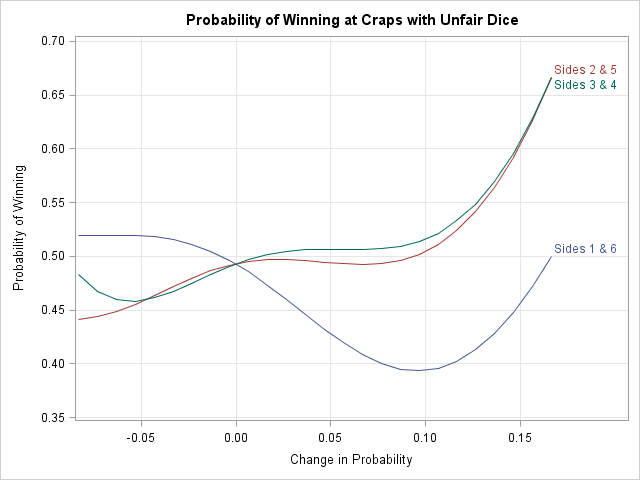The results are shown in the preceding graph (click to enlarge). If you shave the 1 and 6 faces, you dramatically decrease the probability of the shooter winning. If you shave the 3 and 4 faces, you increase the probability, but the effect is not as dramatic. Presumably "the house" would want to shave the 1 and 6 sides, whereas a crooked shooter would want to try to replace the house dice with dice that are shaved on the 3 and 4 faces. However, to increase the probability of the shooter winning by, say, 5%, the 3-4 faces would need to be shaved by a lot. In contrast, to decrease the probability of the shooter winning by 5%, much less tampering is required of the 1-6 faces. Thus the "house" that cheats is less likely to be detected than the shooter that cheats!

Here's the program that creates the graph:

```/* change probability of landing on 1-6, 2-5, or 3-4 sides */ /* Idea from J. C. Wang: http://www.stat.wmich.edu/wang/667/egs/craps2.html */ shaved = T( do( -1/12, 1/6, 0.01 ) ); WinProb = j(nrow(shaved), 3); /* results: winning probability */ do side = 1 to 3; do i = 1 to nrow(shaved); dieProb = j(1, 6, 1/6 - shaved[i] ); dieProb[side||7-side] = 1/6 + 2*shaved[i]; WinProb[i, side] = CrapsWin(dieProb); end; end;   /* graph the data with PROC SGPLOT */ A = shaved || WinProb; create Craps from A[c={"Shaved" "Side1" "Side2" "Side3"}]; append from A; close Craps; quit;   proc sgplot data=Craps; title "Probability of Winning at Craps with Unfair Dice"; series x=Shaved y=Side1 / curvelabel="Sides 1 & 6"; series x=Shaved y=Side2 / curvelabel="Sides 2 & 5"; series x=Shaved y=Side3 / curvelabel="Sides 3 & 4"; yaxis grid values=(0.35 to 0.7 by 0.05) label="Probability of Winning"; xaxis grid label="Change in Probability"; run;```

I really like this graph because it reveals how important various pairs of faces are to making a point.

For example, a pair of dice that rolls many 1s and 6s are bad for the shooter, because the most common sums will be 2, 7, and 12. You lose if 2 or 12 appear on the first roll, although you win with a 7. However, if you happen to establish a point, you are in trouble. Rolling a 2 or 12 doesn't help you make your point, so the most likely scenario is that you will roll a 7 and lose.

In contrast, a pair of dice that rolls many 3s and 4s is good for the shooter. The most common sums will be 6, 7, and 8. You win with a 7. If you establish a point, it is likely to be a 6 or 8, which are the easiest points to make.

The ideas discussed in this article also apply to other kinds of "crooked dice." The SAS/IML program opens up a variety of scenarios to simulate. For example, if only one face appears more than the others, which face would the shooter want it to be? Which face would the house want it to be? You could also modify the SAS/IML function to take two probability vectors, one for each die. Have fun simulating these scenarios, but remember: no cheating in real life!Gambling games that use dice, such as the game of "craps," are often used to demonstrate the laws of probability. For two dice, the possible rolls and probability of each roll are usually represented by a matrix. Consequently, the SAS/IML language makes it easy to compute the probabilities of various events.

For two fair dice, the probability of any side appearing is 1/6. Because the rolls are independent, the joint probability is computed as the product of the marginal probabilities. You can also create a matrix that summarizes the various ways that each particular number can occur:

```proc iml; dieProb = j(1, 6, 1/6); /* each die is fair: Prob(any face)=1/6 */ /* joint prob is indep = product of marginals */ probs = dieProb` * dieProb; /* 6x6 matrix; all elements = 1/36 */ events = repeat(1:6, 6) + T(1:6); print events[c=("1":"6") r=("1":"6")];```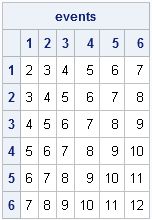You can use the event and probs matrices to compute the probabilities of each possible sum of two dice. There are several ways to do this, but I like to use the LOC function to find the elements of the event matrix that correspond to each roll, and then add the associated probabilities:

```P = j(1, 12, 0); /* probability of each event */ do i = 2 to ncol(P); idx = loc( events=i ); P[i] = sum( probs[idx] ); end; print P[format=6.4 c=("P1":"P12")];```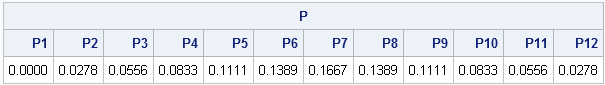The preceding table shows the probabilities of each roll. (Note that the probability of rolling a 1 with 2 dice is zero; the P element is not used for any computations.) You can use the table to compute the probability of winning at craps. If you roll a 7 or 11 on the first roll, you win. If you roll a 2, 3, or 12, you lose. If you roll a 4, 5, 6, 8, 9, or 10 on the first roll (called "the point"), you continue rolling. You win if you can successfully re-roll your "point" before you roll a 7. It is an exercise in conditional probability that you can compute the probability of making a "point" in craps by summing a ratio of probabilities, as follows:

```win = {7 11}; lose = {2 3 12}; point = (4:6) || (8:10);   Pwin1 = sum( P[win] ); /* Prob of winning on first roll */ PLose1 = sum( P[lose] ); /* Prob of losing on first roll */ /* Prob winning = Pr(7 or 11) + Prob(Making Point) */ Pwin = P + P + sum(P[point]##2 / (P[point] + P)); print Pwin1 PLose1 Pwin;```According to the computation, the probability of winning at craps is almost—but not quite!—50%.

This exercise shows that having a matrix and vector language is useful for computing with probabilities. More importantly, however, this post sets the foundations for looking at the interesting case where the two dice are not fair. If you change the definition of dieProb (on the first line of the program) so that it is no longer a constant vector, all of the computations are still valid!

Next week I will post an article that shows how the odds change if some sides of the dice are more likely to appear than others. Feel free to conduct your own investigation of unfair dice before then.